The Numerical Simulation of Properties with High Angular Speed & Low Angular Acceleration in Three and Five Freedoms of Robotic Arm

Journal Name : SunText Review of Material Science

Article Type : Research Article

Authors : Run Xu

Keywords : Numerical simulation; Force and angle; Angular speed and acceleration

Abstract

In robot design and application the force and angle with angular speed is important so this study will model numerical simulation and discuss detail data to investigate their property. The force may increase as arm 1 angle increases whilst it may increase if angular speed increases in three freedoms. Meantime it will decrease if angular acceleration increases. It is found that with the angular speed increasing all three force may increase whilst the angular acceleration will cause its increase too in five freedoms. From these value it is observed that F2 and F1 is prior one to ensure the strength and fatigue life then F3 is second one to estimate its strength whilst F3 may be neglected. The force may increase as arm 1 angle increases whilst it may increase if angular speed increases in three freedoms. Meantime it will decrease if angular acceleration increases. There is big distance to attain 5KN between the conditions. The effective factor turn to the force is F1>F3>F2 in three freedoms. The force may increase from 30N to 18KN and 20KN with F3, F1 and F2 in five freedoms. Among them F3 is the least one and F1 is the biggest one. The effect factor turn is F2 >F1 >F3. So the F2 &F1 is important one while F3 is neglected.

Introduction

In recent the robotic arm has been applied to many occasion in factory which can help people to work in difficult, dirty and dangerous place so its application will be more prevailed in future which is estimated in this study. China has been the largest country which can own the largest domestic market in the world, but it has the third market occupant since it has been not owning the enough advanced condition. So that we must positively construct the clean and criteria house to meet the demand for robotic arm to ensure precise work [1-5]. In robotic design the force is an important factor to consider since the strength must meet demand no matter what it may work in factory. So that according to the function it may be designed to satisfy the no fracture and longer fatigue life for its long life and high load to work in automatic flow line to be substituted to human worker. The biggest one will destination in this paper and how to save manufacture cost is the second one. So it is needed that the mass and load may become first thing to prepare; secondly for the cost decrease the redundant load shall be prohibited. In this paper the condition is changed like angular speed and acceleration to observe the three forces status to ensure security of strength and save cost status. The three freedoms and five ones are investigated in this paper detail with parameters like force and angle with angular speed and acceleration. We try to find the various condition of effective factor in order to search intrinsic properties relationship which is the destination in this paper. In short the properties are searched through parameters in detail. We look forward to finding new change with force and speed & acceleration for further research.

Numerical Simulation

In Figure 1 there are three freedoms in mechanical arm that name as 1~3. Meantime there are two other ones call 4&5which is included in five freedoms as a rotational and crawling function. In Figure 1 the schematic shows the simplified principle of robot. The coordinate XAY is three freedoms and X?A?Y it five freedoms. In this study the five freedoms not three one is deduced since it is complicated (Figure 1,2).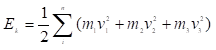Figure 1: construction schematic of mechanical arm in series in robot 3-hand part; 2-wrist part; 1-arm part; 4-waist part; 5-two crawling wheel.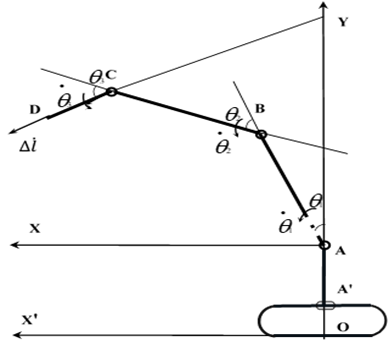Figure 2: Principle schematic of mechanical arm in series in robot.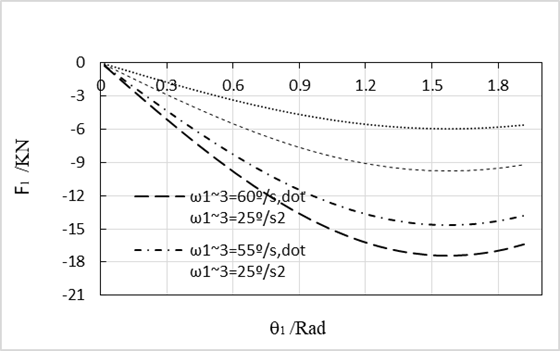(a) F1.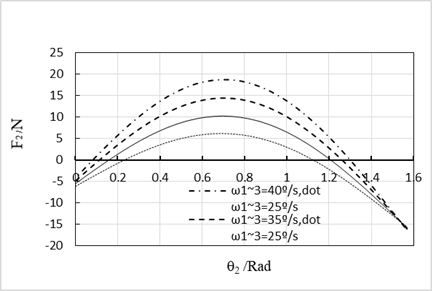(b) F2.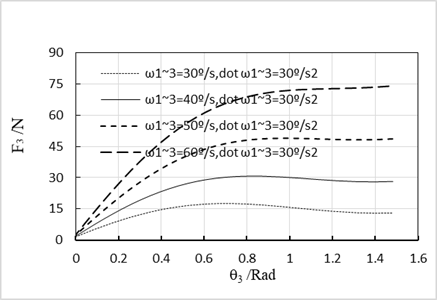Figure 3: The curve of force and angle with various angular speed and acceleration of 25, 30º/s2 in three freedoms of robot arm.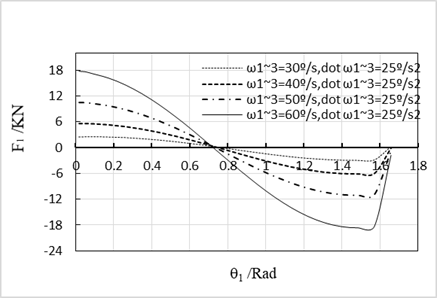(a) F1.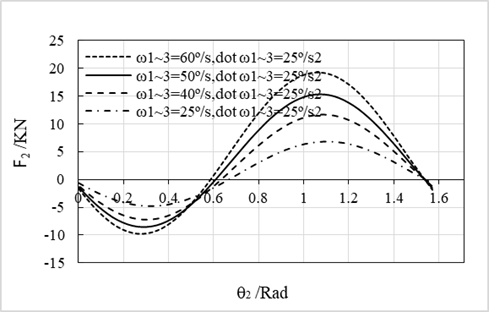(b) F2.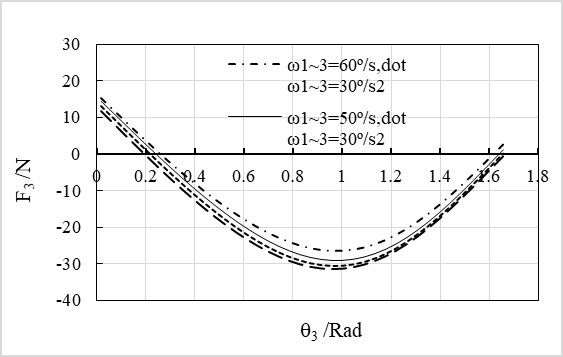Figure 4: The curve of force and angle with various angular speed and acceleration of 25, 30º/s2 in five freedoms of robot arm.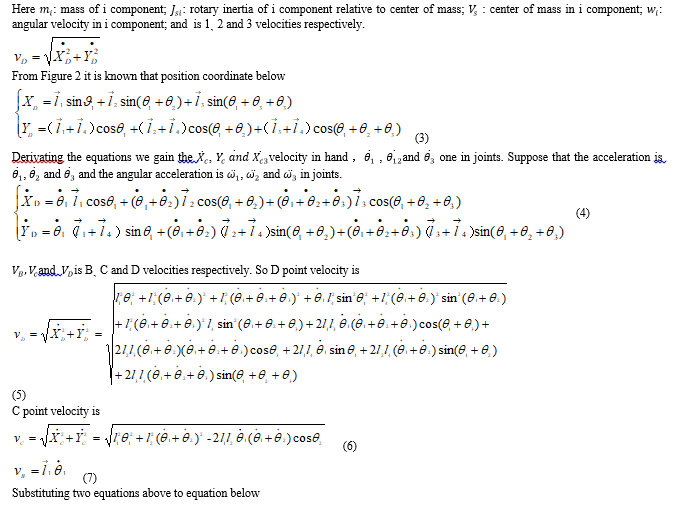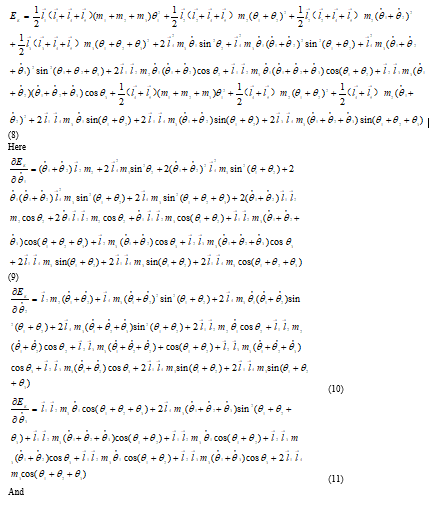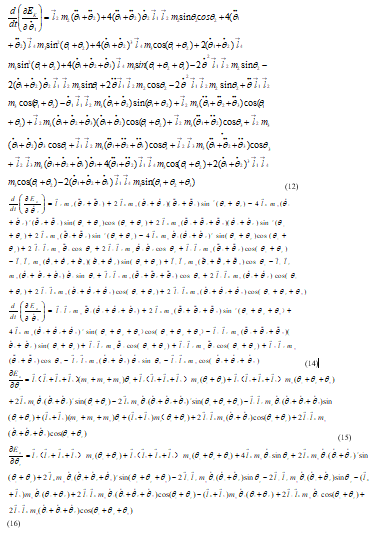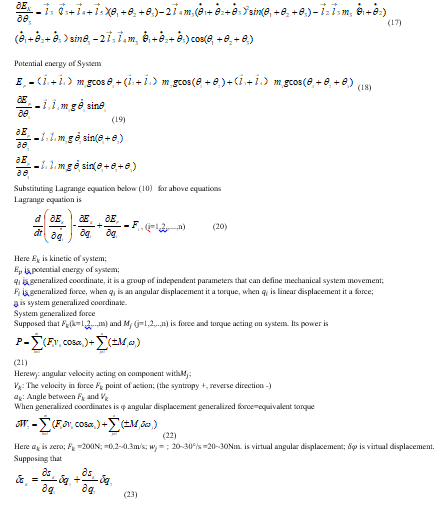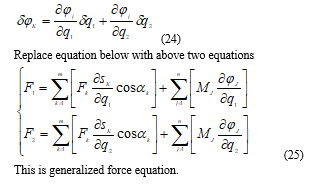Discussions

As seen in Table 1 the parameter in robot arm is listed. [6~7] Here ?1, ?2, ?3 is the arm1, arm2, arm3 angle respectively. l1, l2, l3 is arm length. m1, m2, m3 is arm mass. Number is arm label. According to these parameters the below curves are gained as below in Figure 3 &4. As seen in Figure 3(a~c) the force of arm1 will increase with the angular speed and acceleration increasing that expresses the proportional relation between them fitting to Newton theory well. That says that angular speed raises the acceleration meantime the later raise the force too. They all distributes into sinusoidal continuous wave that forms semiwave with 90º. The force may increase from 30N to 18KN and 20KN with F3, F1 and F2. Among them F3 is the least one and F1 is the biggest one. The effect factor turn is F2 >F1 >F3 in Figure 4 in three freedoms. From these value it is observed that F2 is prior one to ensure the strength and fatigue life then F1 is second one to estimate its strength whilst F3 may be neglected. In contrast to it in Figure 3 the F1 is 18KN which is main parameter to check the robotic arm and F3 is second one and finally F2 is 20N which may be neglected in five freedoms system. The effect turn is F2>F1>F3 here (Table 1).

Table 1: Parameters of robot arms.

 items Value Item Value l1 /m 0.55 /°/s 30~60 l2 /m 0.5 /°/s 30~60 l3 /m 0.3 /°/s 30~60 m1/N 7.7 /°/s2 25,30 m2/N 6.6 /°/s2 25,30 m3/N 4.0 /°/s2 25,30

As seen in Figure 3 the force may increase as arm 1 angle increases whilst it may increase if angular speed increases in three freedoms. Meantime it will decrease if angular acceleration increases in Figure 3(d). The maximum is 18KN in Figure 3(a) if angular speed is 35~60º/s and acceleration is 25~30º/s2 so this point will be checked to ensure the robotic arm strength. There is big distance to attain 5KN between the conditions. The effective factor turn to the force is F1>F3>F2 in three freedoms (Figure 3).

In the modeling of five freedoms in movement of robotic arm the kinetic equation is established according to Lagrange formula based on three freedoms robotic arm. It compensates the blank in four freedoms and one impulsion on robot. It is found that the first and second solution is complicated and long the whole equations is concise than the traditional equation. This is a blank in five freedoms which can shorten the whole numerical computation a lot. Referring to the important occasion the kinetic equation will only be computed on three freedoms according to this study (Figure 4).

It is suggested that the big arm happens when angular speed and acceleration is big. So that the reasonable parameters are chosen to design and estimate their properties is important. Not to choose big angular speed and acceleration is key in order to increase the capability and property that may increase the whole cost as well. Overview the computation is shorter than the five freedoms traditional one. The solution is easy to use in software like Excel and Origin. The result is satisfactory and precise to be adopted to numerical simulation so the five freedoms method based on three freedoms is feasible.

Conclusions

• There is big distance to attain 5KN between the conditions. The effective factor turn to the force is F1>F3>F2 in three freedoms.
• The force may increase from 30N to 18KN and 20KN with F3, F1 and F2 in five freedoms. Among them F3 is the least one and F1 is the biggest one. The effect factor turn is F2 >F1 >F3. So the F2 &F1 is important one while F3 is neglected.

References

1. Zhang CE. Machinery dynamics. Higher Educational Press. 2008; 96
2. Yonglun X, Lixin T. The base of robotic technology. Huazhong University Technology press. 1999; 89-90.
3. Xu R. The dynamic equation on hammer with lagrange in robotic arm. Social Sci learning Edu J. 2020; 5: 297-300.
4. Doyi L, Lei C, Xiaolong S, Zhinchao W, Wei W, Xulong Y, et al. Structure design of pineapple picking manipulator. Agricultural Engg. 2019; 9: 1-2.
5. Xu R, Hur B. A simulation between torque and angle with speed on five freedoms of robot mechanical arm in multi body systems. Saudi J Civil Engg. 2021; 5: 91-93.
6. Yan P. Base on dynamics analysis and precise design with mechanical arm of arthritis rub. Master Degree Thesis, Jiangsu University of Technology. 2012; 3: 18
7. Li Z, Wu C. The dynamics solving method of flexible joint robot based on quantized state system.  J Mechanical Engg. 2020; 56: 122.